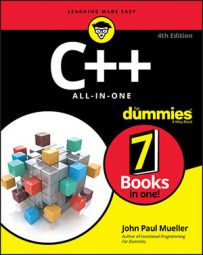##### C++ All-in-One For DummiesComputer applications perform many comparisons. In most cases, you don’t know what the values are in advance or you wouldn’t be interested in performing the comparison in the first place. The min() and max() functions make it possible to look at two values and determine the minimum or maximum value.

The MinAndMax example demonstrates how you use these two functions:

```#include <iostream>
using namespace std;
int main()
{
int Number1, Number2;
cout << "Type the first number: ";
cin >> Number1;
cout << "Type the second number: ";
cin >> Number2;
cout << "The minimum number is: " << min(Number1, Number2) << endl;
cout << "The maximum number is: " << max(Number1, Number2) << endl;
return 0;
}```

In this case, the code accepts two numbers as input and then compares them using min() and max(). The output you see depends on what you provide as input, but the first output line tells you which number is smaller and the second tells you which is larger. Assuming you provide values of 5 and 6, here is the application output you see:

```Type the first number: 5
Type the second number: 6
The minimum number is: 5
The maximum number is: 6```